# Demonstration of SUP Clustering

This document demonstrates the use of supc package for clustering. We illustrate the codes to perform the self-updating process (SUP), the parameter selection and the clustering result visualization. Two artificial clustering benchmark data sets and a gene expression data are included. We use the three data sets as examples of (i) data with clusters of varying sizes, densities and shapes, (ii) data with many clusters and (iii) data with a substantial level of noises and outliers. These are the three types of data which SUP has been shown to be effective in clustering.

``library(supc)``

# 1 Example 1 - Data that has clusters of different sizes, densities and shapes

The first artificial data was introduced in the paper that proposed the CURE clustering algorithm by Guha, Rastogi, and Shim (2001). The data was generated to have five clusters: one big circle, two small circles, and two ellipses. Due to the large differences in sizes and shapes of the clusters, the challenge is to identify and distinguish between the five clusters, while most of the clustering algorithms failed. In addition, some noise data points were generated to connect the two ellipses, and some were randomly scattered. The purpose of these noise data points, according to the authors, were to demonstrate that CURE is insensitive to outliers. The codes that generate this example data are provided in the reference manual.

``````data(shape)
x=as.matrix(shape[,1:2])
plot(x, main="Example 1 Data")``````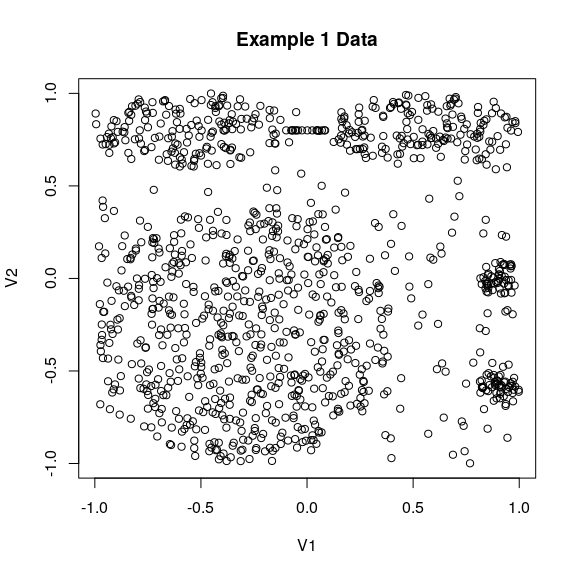The self-updating process has two parameters: the influential range r and the temperature T. Throughout this document we use the function default setting for dynamic temperature, that makes r the only parameter to be determined.

Shiu and Chen (2016) proposed to select the value of parameter r on the basis of the valleys of the frequency polygon of the pairwise distances between the samples to be clustered. Here we plot the frequency polygon using 100 bands. We can further use `diff(x.freq\$count)<0` to identify the band which has a smaller frequency count than the band preceding it. This helps to find the locations of the valleys. The following plot shows the frequency polygon of the pairwise distances and the valley locations.

``````x.freq <- freq.poly(x, breaks = 100)
tmp=cbind(dist=x.freq\$mids[-1], freq=x.freq\$count[-1], diff=diff(x.freq\$count))
tmp[tmp[,3]<0,][1:5,]``````
``````##      dist freq diff
## [1,] 0.51 6229  -56
## [2,] 0.73 7695  -69
## [3,] 0.81 8531  -30
## [4,] 0.85 8565  -83
## [5,] 0.89 8669  -73``````
``abline(v=c(0.51, 0.73, 0.85), lty=2)``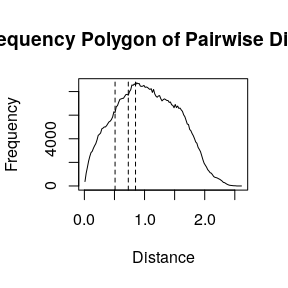Our experience shows that the use of valley values very often produces good clustering results. For this example data, we select the influential range r to be the previously identified valley values to perform SUP clustering.

``x.supcs <- supc1(x, r=c(0.40, 0.51, 0.73, 0.85), t = "dynamic", implementation = "R")``

The following plots show the results of SUP clustering using each selected value of r, respectively. The plots also show that the cluster size and the number of clusters decrease with the use of a larger r value. The five-cluster-structure of this data is successfully identified when we take r to be the valley values of 0.51 and 0.73.

``````oldpar <- par(mfrow=c(2,2))
tryCatch({
for (i in 1:4) plot(x, col=x.supcs\$cluster[[i]], main=paste("r=", x.supcs\$r[[i]], sep=""))
}, finally = {
par(oldpar)
})``````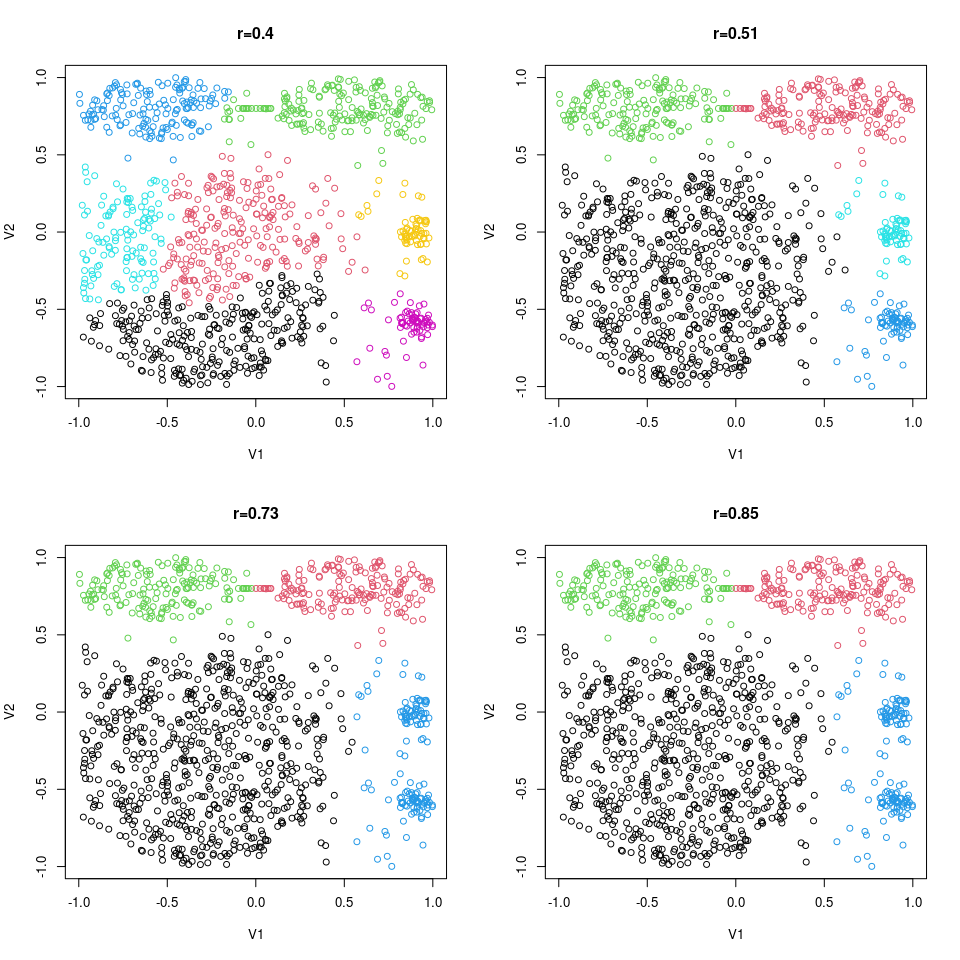# 2 Example 2 - Data that has many clusters

The second dataset was introduced in the paper by Veenman, Reinders, and Backer (2002). The data was generated to consist of as many as 31 randomly placed Gaussian clusters. Clustering such datasets is difficult for the partition type of clustering algorithms that require an initial set, because proper selections of the initial sets are crucial to prevent these algorithms from trapping into a local minimum, which may consequently lead to poor clustering results.

``````data(D31)
x=as.matrix(D31[,1:2])
plot(x, main="Example 2 Data")``````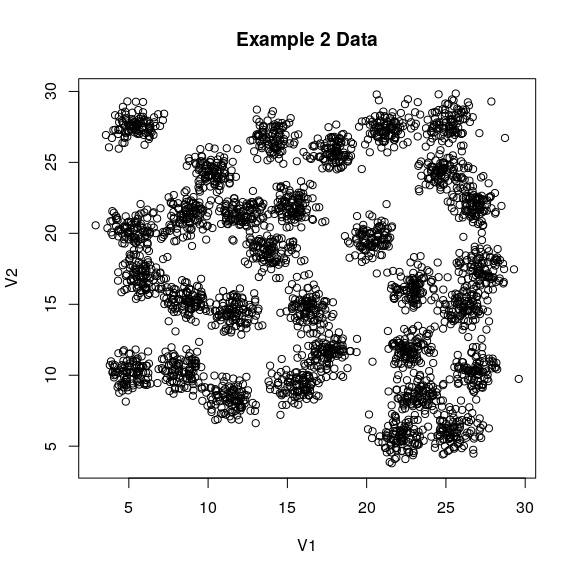``````x.freq <- freq.poly(x, breaks = 100)
tmp=cbind(dist=x.freq\$mids[-1], freq=x.freq\$count[-1], diff=diff(x.freq\$count))
tmp[tmp[,3]<0,][1:5,]``````
``````##      dist   freq  diff
## [1,] 1.75  42674 -3619
## [2,] 2.25  41803  -871
## [3,] 7.75 131250 -3238
## [4,] 8.25 121445 -9805
## [5,] 8.75 114197 -7248``````
``abline(v=2.25, lty=2)``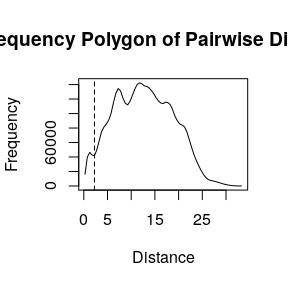The frequency polygon shows a valley at pairwise distance=2.25. Using this value, SUP produces 34 clusters, among which are three singleton clusters, each consists of only a single data point.

``````x.supcs <- supc1(x, r =2.25, t = "dynamic", implementation = "R")
table(x.supcs\$cluster)``````
``````##
##   1   2   3   4   5   6   7   8   9  10  11  12  13  14  15  16  17  18  19  20
## 106 105 105 104 104 102 101 101 101 101 101 101 101 101 100 100 100 100 100 100
##  21  22  23  24  25  26  27  28  29  30  31  32  33  34
##  99  99  99  98  97  97  96  96  95  95  92   1   1   1``````

We plot the cluster numbers at the centers of each cluster to present the clustering results. The cluster numbers of the three singleton clusters are denoted with red color instead of black. From the plot, we can see that the three singleton clusters are in fact three outlier data points.

``````plot(x, col=adjustcolor(x.supcs\$cluster, alpha.f=0.2),
main=paste("r=",x.supcs\$r, sep=""))
text(x=x.supcs\$centers[1:31,], labels=1:31, cex=1.2)
text(x=x.supcs\$centers[32:34,], labels=32:34, col=2)``````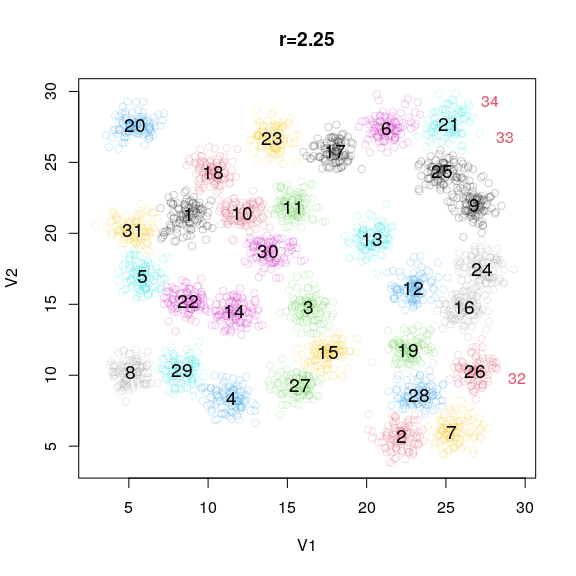This result shows that SUP correctly identifies the 31 major clusters. SUP also identifies three data points as outliers, by forming them as isolated singleton clusters. If the user does not wish to separate out the outliers and the noise data points, two approaches can be taken: (i) merge each isolated tiny cluster to the closest major cluster, (ii) use a larger r value to extend the sizes of the major clusters, so that the outliers and the noise data points may be included.

# 3 Example 3 - Gene expression data

This example uses the gene expression data presented in Golub et al. (1999). We obtained the data from the multtest package in Bioconductor. We furthre normalized the expression values by genes to ensure an equal weight of each gene. In this example, we demonstrate the use of SUP in discovering gene cluster patterns in the presence of scattered genes.

The following plot shows the frequency polygon of the pairwise distances between genes. In situations when there is no valley in the plot, we recommend the user to perform preliminary clusterings using the function default `rp` values, which covers a wide range from 0.0005 to 0.3. From there, subsequent changes of the r value can be made to achieve a better the clustering result.

``````data(golub)
x=golub
x.freq <- freq.poly(x, breaks = 100)``````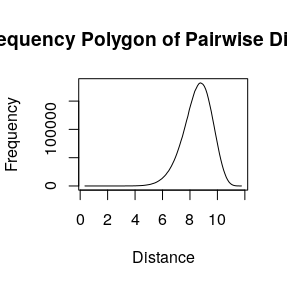SUP using the first default `rp` value produces 1930 clusters, among which only five clusters have sizes larger than 50. There are 1866 clusters which have a single gene.

``````x.supcs <- supc1(golub, t="dynamic", implementation = "R")
max(x.supcs[]\$cluster)``````
``##  1930``
``table(x.supcs[]\$size)``
``````##
##    1    2    3    4    5    6    7    8   10   11   12   13   27   47   59   88
## 1866   32    9    3    2    2    4    1    1    1    1    1    1    1    1    1
##  143  201  413
##    1    1    1``````

In this example we use heatmaps to present SUP clustering results. Heatmaps are a very useful tool to visualize the cluster patterns and the structure of the data, especially when the dimension of the data is greater than three. The supc package therefore provides the `type="heatmap"` option in the `plot` function, that facilitates the user to create heatmaps based on the clustering results. In addition, the `ColorRampPalette` function and the `image.plot` function from `library(fields)` can be used to create color legends for the heatmap.

``````qt=seq(-3, 3, by=0.05)
bluered=colorRampPalette(c("blue","white","red"))(length(qt)-1)``````

The following three heatmaps show the patterns of the identified gene clusters obtained from SUP using the first three default `rp` values, respectively. Take the first heatmap as an example, that presents the clustering results using `rp=0.0005`, corresponding to a r value of 4.335. The resulting 1930 clusters are displayed by their sizes in the order from left to right. On the left of this heatmap shows the cluster patterns of the largest five clusters. The sizes of the clusters are denoted on top of each cluster, which are 413, 201, 143, 88 and 59, respectively. On the middle to the right of this heatmap shows the 1866 genes of singleton clusters. This heatmap demonstrates the strength of SUP that can isolate the noise data points without disturbing the clustering process and influencing the final clustering results.

``library(fields)``
``## Loading required package: spam``
``## Loading required package: dotCall64``
``## Loading required package: grid``
``````## Spam version 2.7-0 (2021-06-25) is loaded.
## Type 'help( Spam)' or 'demo( spam)' for a short introduction
## and overview of this package.
## Help for individual functions is also obtained by adding the
## suffix '.spam' to the function name, e.g. 'help( chol.spam)'.``````
``````##
## Attaching package: 'spam'``````
``````## The following objects are masked from 'package:base':
##
##     backsolve, forwardsolve``````
``## Loading required package: viridis``
``## Loading required package: viridisLite``
``````## See https://github.com/NCAR/Fields for
##  an extensive vignette, other supplements and source code``````
``````par(mfrow=c(3,1), oma=c(1,0,3,0))
for (i in 1:3){
plot(x.supcs[[i]], type="heatmap", breaks=qt, col=bluered, major.size=50,
xlab="Genes", ylab="Samples")
image.plot(x, breaks=qt, col=bluered,
legend.only=TRUE, horizontal=TRUE, legend.mar=0, smallplot=c(0.7, 0.95, 0.16, 0.20))

}``````